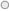# Optimize SUMMARIZE with ADDCOLUMNS in Dax #ssas #tabular #dax #powerpivotIf you started using DAX as a query language, you might have encountered some performance issues by using SUMMARIZE. The problem is related to the calculation you put in the SUMMARIZE, by adding what are called extension columns, which compute their value within a filter context defined by the rows considered in the group that the SUMMARIZE uses to produce each row in the output.

Most of the time, for simple table expressions used in the first parameter of SUMMARIZE, you can optimize performance by removing the extended columns from the SUMMARIZE and adding them by using an ADDCOLUMNS function. In practice, instead of writing